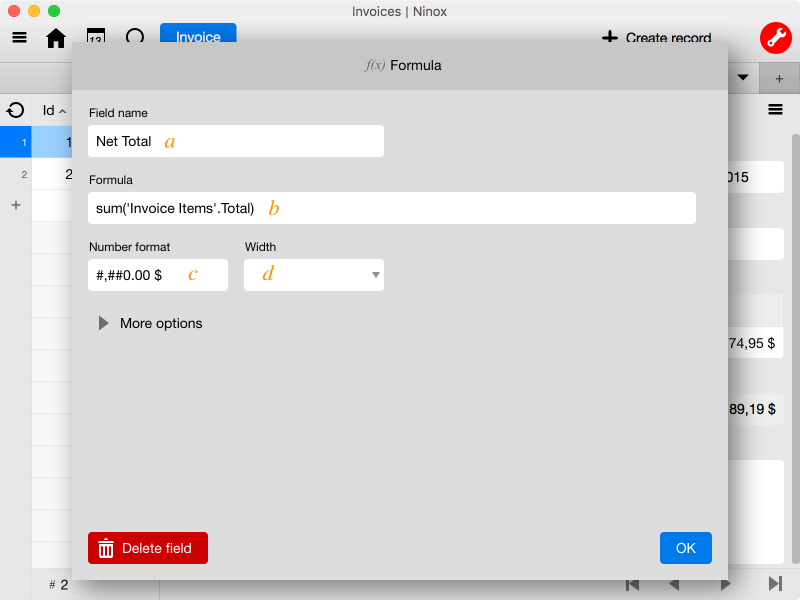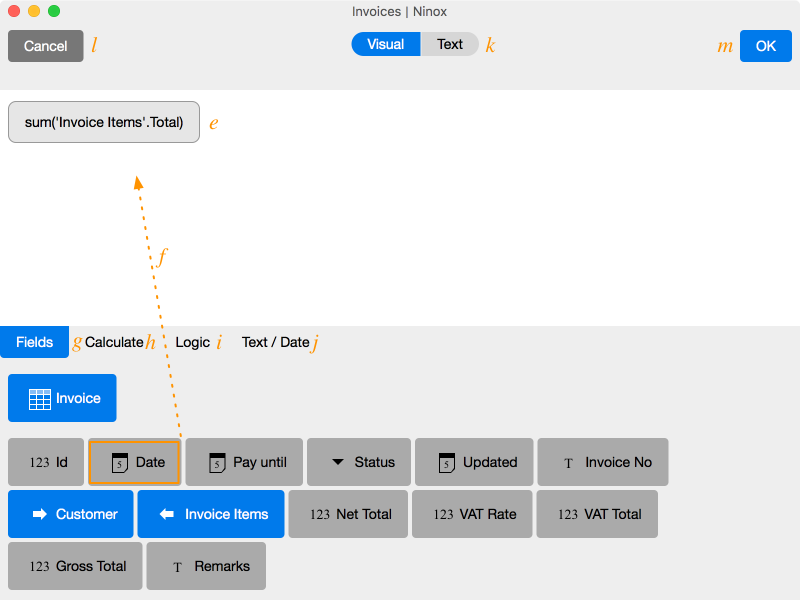## Formulas

Ninox uses formulas in different situations. The following screenshot shows how to define a formula field in a table.a) Like any other field, a formula field has got a name that is shown in the form or as a table column.

b) Tap on the field ‘Formula’ to open the formula editor.

c) If the formula returns a numeric value, you can set a number format for the result. This way, you can have the number displayed as an amount of money, for example.

d) Also, you can determine the field width of the formula in the form.

The Formula Editor

The formula editor opens on the lower screen half. It is divided into three areas: the big white field contains the inserted formula, followed by a list of elements that you can add to the formula: data fields, calculations and so on. If the formula is incomplete or incorrect, there will be a warning above the formula.

Drag the elements you want to add from below into the white formula field. The position where the element is about to be integrated will light up in red.In order to delete the element, drag it from the white field upwards..

e) The formula field

f) Add new elements using drag & drop.

l) Cancels the editing process of the formula

m) Confirms the entered formula. The entry can only be confirmed, as long as there is no formula error marked.

k) You can also use the text editor to type in formulas with Ninox language.

Formula Elements

g) Fields

Among ‘fields’ there are all the fields of the active table – which are relevant for formulas. The first row is the table name, below there are the data fields.

Table references are special: they are shown in blue; you can’t drag them directly into the formula. Tap on the link instead. Now, all relevant fields from the referenced table are shown. In the first row you can navigate back.

If you follow a reverse reference (<=), then you won’t be able to drag their data fields directly into the formula (also blue). Tap on the data field to obtain a list of possible aggregations: sum, average, minimum, maximum, count etc.

h) Calculate

The tab ‘Calculate’ comprehends all available mathematical operations, as well as an entry field for constant numerical values (1234).

• Reference of the mathematical functions

i) Logic

Under the tab ‘Logic’ you can find functions for conditioned calculations (if / then / else), logical references and comparative operators.

• Reference of the logical functions

j) Text / Date

Text-related and date-related functions are filed under the tab ‘Text / Date’. When you calculate with dates, please keep in mind the special abilities of the operators +,-,*,/. Use ‘+’ to concatenate strings.

• Reference of the text and date functions